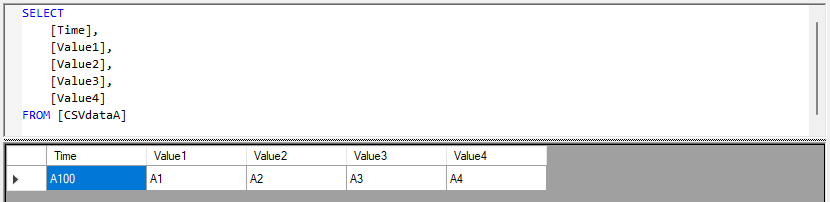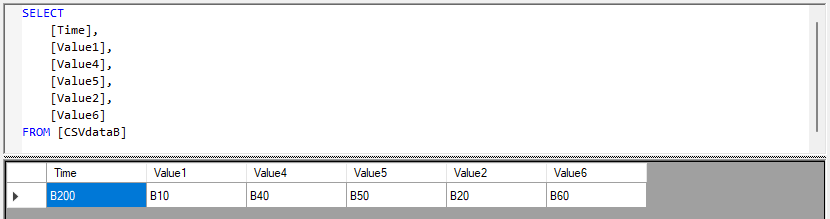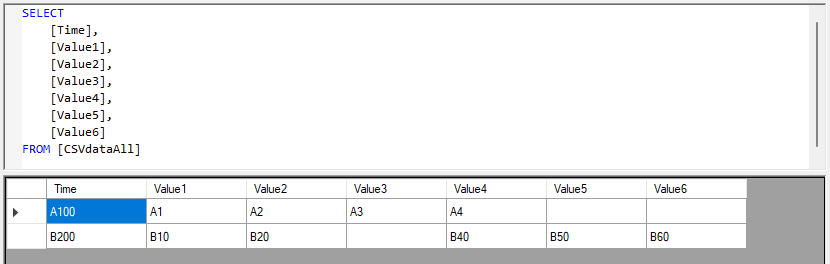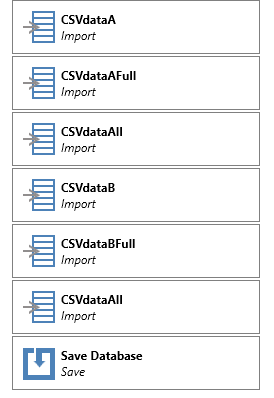# Combining two tables with different columns

In this example I have two tables with different number of columns. Some columns do exist in both tables, but not necessarily in the same column position.

Table A:Table B (notice the "wrong" position of Value2):Desired combination of the tables A and B:To recreate, create an InMemory project with a CSV data source (in my example, the original sources for Table A and Table B are two CSV files).

InMemory project, visual:InMemory project, code:

`LOAD ASSEMBLY 'TARGIT.Csv.dll' //serverDATASOURCE [CSV] = DOTNET CONNECTION 'TARGIT.CSV.CsvConnection' 'HasHeaders=true;loadasstring=false;type=local;delimiter=,;encoding=Windows-1252;culture=en-US;detectionrowscount=100;skiplines=0;'IMPORT [CSVdataA] = [CSV].{SELECT * FROM [C:\Temp\CSVdata\CSVdataA.txt]}IMPORT [CSVdataAFull] = [ME].{SELECT CAST(COLUMNEXISTS(Time,Time,null) as VARCHAR) AS Time,CAST(COLUMNEXISTS(Value1,Value1,null) as VARCHAR) AS Value1,CAST(COLUMNEXISTS(Value2,Value2,null) as VARCHAR) AS Value2,CAST(COLUMNEXISTS(Value3,Value3,null) as VARCHAR) AS Value3,CAST(COLUMNEXISTS(Value4,Value4,null) as VARCHAR) AS Value4,CAST(COLUMNEXISTS(Value5,Value5,null) as VARCHAR) AS Value5,CAST(COLUMNEXISTS(Value6,Value6,null) as VARCHAR) AS Value6FROM [CSVdataA]}IMPORT [CSVdataAll] = [ME].{SELECT * FROM [CSVdataAFull]}IMPORT [CSVdataB] = [CSV].{SELECT * FROM [C:\Temp\CSVdata\CSVdataB.txt]}IMPORT [CSVdataBFull] = [ME].{SELECT CAST(COLUMNEXISTS(Time,Time,null) as VARCHAR) AS Time,CAST(COLUMNEXISTS(Value1,Value1,null) as VARCHAR) AS Value1,CAST(COLUMNEXISTS(Value2,Value2,null) as VARCHAR) AS Value2,CAST(COLUMNEXISTS(Value3,Value3,null) as VARCHAR) AS Value3,CAST(COLUMNEXISTS(Value4,Value4,null) as VARCHAR) AS Value4,CAST(COLUMNEXISTS(Value5,Value5,null) as VARCHAR) AS Value5,CAST(COLUMNEXISTS(Value6,Value6,null) as VARCHAR) AS Value6FROM [CSVdataB]}IMPORT [CSVdataAll] = [ME].{SELECT * FROM [CSVdataBFull]}SAVE`